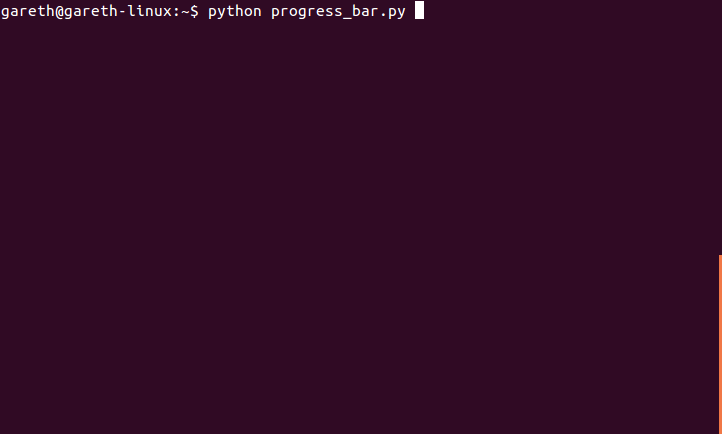## Saturday, 26 July 2014

### Pretty Python ProgressOften when I write loops in Python I want to how much progress has been made. A simple way is to put a counter and a print statement:
``````import time
progress = 0
thingsToProcess = range(543)
for thingToProcess in thingsToProcess:
progress += 1
print "%s/%s" % (progress, len(thingsToProcess))
time.sleep(0.05)
``````
Or in fewer lines:
``````import time
thingsToProcess = range(543)
for progress, thingToProcess in enumerate(thingsToProcess):
print "%s/%s" % (progress, len(thingsToProcess))
time.sleep(0.05)
``````
Which is OK, but it spams the output screen with a line for each iteration. We can improve a little bit by only printing on every Nth iteration:
``````import time
thingsToProcess = range(543)
for progress, thingToProcess in enumerate(thingsToProcess):
if progress % 10 == 0:
print "%s/%s" % (progress, len(thingsToProcess))
time.sleep(0.05)
``````
But we can do even better with not too much effort. Let's build progress bars and percentage counters! We use ANSI codes to print nice(?) colours in the terminal, and the "\r" special character (carriage return) to print over the same line in the terminal. We also need to "flush" the standard output on each print, or it will get buffered automatically and we'll only see the final line. Note also the comma at the end of the print statement in print_progress - this suppresses the newline character. To use, just call the print_progress function from inside any loop where:
• You know the index of the current iteration
• You know how many iterations there will be in total
• There are no other print statements in the loop
Just change the string argument "colour" ("cyan") to any of the colours in the dictionary defined in print_progress to see the progress in other nice(?) colours. By subtracting 60 from each number in the colour dictionary a different shade of that colour will be printed instead (e.g. try using "32" instead of "92" for Green).
``````import sys
import time

def print_progress(current, total, colour=""):
current += 1 # be optimistic so we finish on 100
colours = {"":0, "black":90, "red":91, "green":92, "yellow":93, "blue":94, "purple":95, "cyan":96, "white":97}
COLOUR_START = '\033[%sm' % (colours.get(colour))
COLOUR_END = '\033[0m'
percent_float = float(current)/float(total) * 100
percent = "%.1f" % percent_float
bar = "|%s>%s|" % ("-" * int(percent_float/4), " " * (25 - int(percent_float/4)))
print "\r%s%s / %s - %s%% %s %s" % (COLOUR_START, current, total, percent, bar, COLOUR_END),
sys.stdout.flush()

thingsToProcess = range(145)
for progress,value in enumerate(thingsToProcess):
print_progress(progress, len(thingsToProcess), "cyan")
time.sleep(0.05)``````

Edit: I updated code, which adds time remaining and uses a Class. Less hacky, more efficient, better. See demo function for example usage. Full listing below.

``````# Gareth Dwyer, 2014
# A simple progress bar for Python for loops, featuring
#   * Percentage counter
#   * ASCII bar
#   * Time remaining
#   * Customizable additional info (display last data processed)
#   * Customizable colours
#

import sys
import time
from datetime import datetime, timedelta

def convert_seconds(num_seconds):
""" convert seconds to days, hours, minutes, and seconds, as appropriate"""
sec = timedelta(seconds=num_seconds)
d = datetime(1,1,1) + sec
return ("%dd %dh %dm %ds" % (d.day-1, d.hour, d.minute, d.second))

def run_demo():
""" create a ProgressBar and run """
pb = ProgressBar("cyan")
data = range(30,56)
for i,v in enumerate(data):
time.sleep(0.5)
pb.print_progress(i, len(data), v)

class ProgressBar:

def __init__(self, colour="green"):
""" Create a progress bar and initalise start time of task """
self.start_time = time.time()
self.colours = {"":0, "black":90, "red":91, "green":92, "yellow":93, "blue":94, "purple":95, "cyan":96, "white":97}
self.start_colour = "\033[%sm" % (self.colours.get(colour))
self.end_colour = "\033[0m"

""" Call inside for loop, passing current index and total length of iterable """
current += 1 # be optimistic so we finish on 100
percent = float(current)/float(total) * 100
remaining_time = convert_seconds((100 - percent) * (time.time() - self.start_time)/percent)
percent_string = "%.1f" % percent
bar = "|%s>%s|" % ("-" * int(percent/4), " " * (25 - int(percent/4)))
print "\r%s%s / %s - %s%% %s %s remaining: %s %s" % (self.start_colour, current, total, percent_string, bar, additional_info, remaining_time, self.end_colour),
sys.stdout.flush()

if __name__ == '__main__':
run_demo()
``````